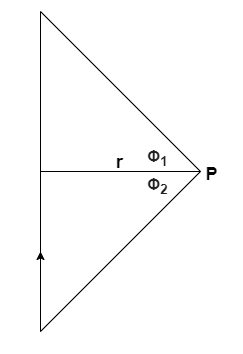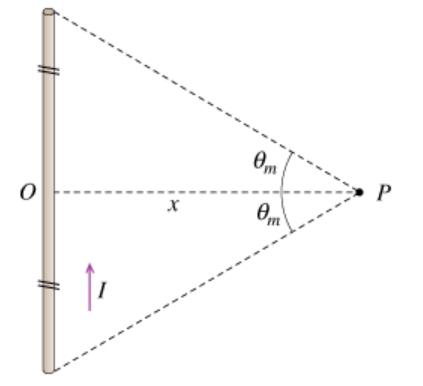# Problem: A steady current I is flowing through a straight wire of finite length.Part AFind B1, the magnitude of the magnetic field generated by this wire at a point P located a distance x from the center of the wire. Assume that at P the angle subtended from the midpoint of the wire to each end is θm as shown in the diagram (Figure 1).

###### FREE Expert Solution

The magnetic field at point P due to a finite wire carrying current:In this problem:

Φ1 = θm

Φ2 = θm

84% (45 ratings)###### Problem Details

A steady current I is flowing through a straight wire of finite length.Part A

Find B1, the magnitude of the magnetic field generated by this wire at a point P located a distance x from the center of the wire. Assume that at P the angle subtended from the midpoint of the wire to each end is θm as shown in the diagram (Figure 1).